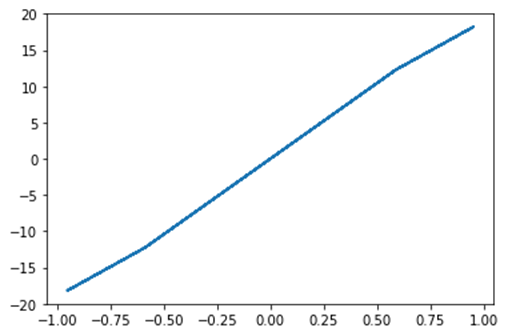Home » Blog » Optimization and Fit in SciPy – scipy.optimize

# Optimization and Fit in SciPy – scipy.optimize

## Optimization and Fit in SciPy – scipy.optimize

• Optimization provides a useful algorithm for minimization of curve fitting, multidimensional or scalar and root fitting.
• Let’s take an example of a Scalar Function, to find minimum scalar function
```%matplotlib inline
import matplotlib.pyplot as plt
from scipy import optimize
import numpy as np

def function(a):
return   a*2 + 20 * np.sin(a)
plt.plot(a, function(a))
plt.show()
#use BFGS algorithm for optimization
optimize.fmin_bfgs(function, 0)```

Output:Optimization terminated successfully.

Current function value: -23.241676

Iterations: 4

Function evaluations: 18

array([-1.67096375])

• In this example, optimization is done with the help of the gradient descent algorithm from the initial point
• But the possible issue is local minima instead of global minima. If we don’t find a neighbor of global minima, then we need to apply global optimization and find global minima function used as basinhopping() which combines local optimizer.

optimize.basinhopping(function, 0)

Output:

```fun: -23.241676238045315
lowest_optimization_result:
fun: -23.241676238045315
hess_inv: array([[0.05023331]])
jac: array([4.76837158e-07])
message: 'Optimization terminated successfully.'
nfev: 15
nit: 3
njev: 5
status: 0
success: True
x: array([-1.67096375])
message: ['requested number of basinhopping iterations completed successfully']
minimization_failures: 0
nfev: 1530
nit: 100
njev: 510
x: array([-1.67096375])```